Get instant live expert help with Excel or Google Sheets“My Excelchat expert helped me in less than 20 minutes, saving me what would have been 5 hours of work!”

#### Post your problem and you’ll get Expert help in seconds.

Your message must be at least 40 characters
Our professional Expert are available now. Your privacy is guaranteed.

# Excel COLUMNS Function

While working with Excel, we are able to determine the number of columns of any cell or range by using the COLUMNS function.  This step by step tutorial will assist all levels of Excel users in the usage and syntax of the COLUMNS function.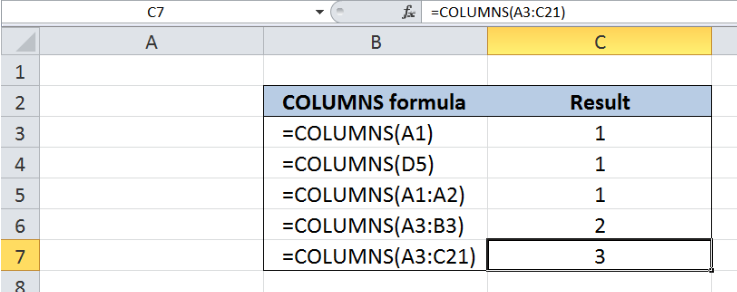Figure 1. Final result: Excel COLUMNS function

## Syntax of the COLUMNS function

COLUMNS function returns the number of columns in an array

`=COLUMNS(array)`

• array – reference to a range of cells whose number of columns we want to determine; could be an array, a formula or reference to a range of cells

## Setting up our Data

Our data consists of two columns: COLUMNS formula (column B) and Result (column C).  We want to enter the COLUMNS formulas in column B and see the results in column C.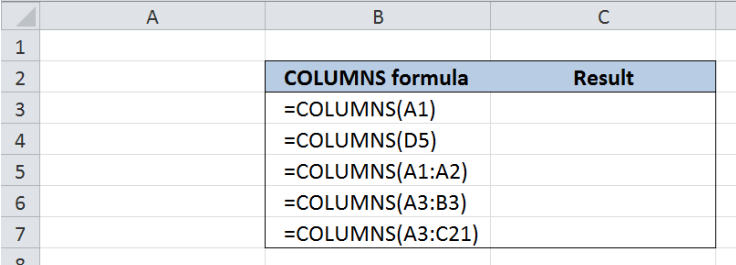Figure 2. Sample data to determine the number of columns

## Determine the number of columns of any cell

In order to determine the number of columns of a certain cell, we enter the cell reference as the argument in our COLUMNS function.  Let us follow these steps:

Step 1.  Select cell C3

Step 2.  Enter the formula: `=COLUMNS(A1)`

Step 3.  Press ENTER

As a result, the value in cell C3 is 1, because cell A1 is a single cell occupying only one column in the worksheet.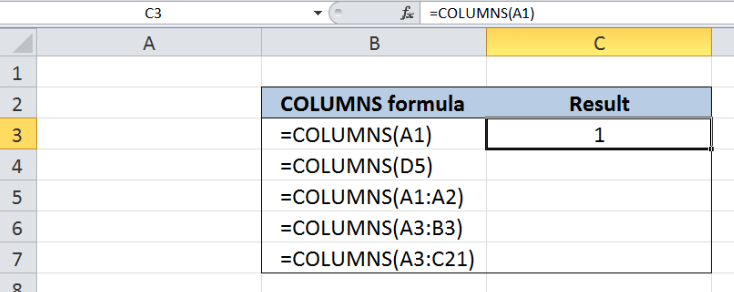Figure 3.  Using COLUMNS function to determine number of columns of cell A1

In the same way, when we enter another cell as the argument in our COLUMNS function, we will obtain the same result.  Let us take another example:

Step 1.  Select cell C4

Step 2.  Enter the formula: `=COLUMNS(D5)`

Step 3.  Press ENTER

As a result, the value in cell C4 is 1, because cell D5 is also a single cell occupying only one column in the worksheet.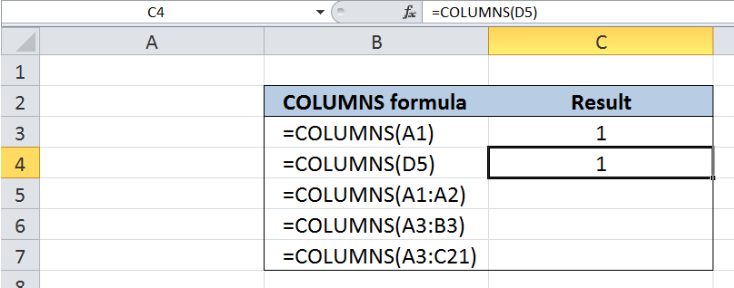Figure 4.  Using COLUMNS function to determine number of columns of cell D5

## Determine the number of columns in a range

In order to determine the number of columns of a given range using the COLUMNS function, let us follow these steps:

Step 1.  Select cell C5

Step 2.  Enter the formula: `=COLUMNS(A1:A2)`

Step 3.  Press ENTER

The range A1:A2 is our reference for the COLUMNS function.  The resulting value in cell C5 is also 1, because our range refers to two cells occupying only one column, which is column A.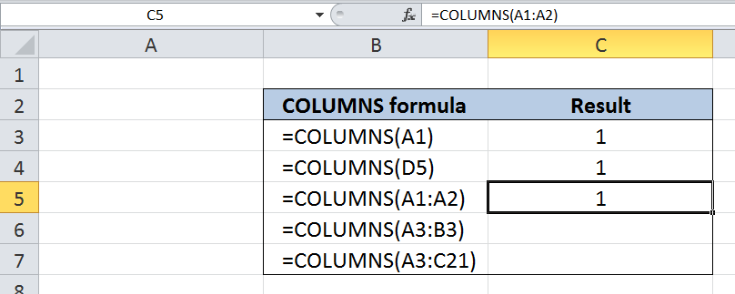Figure 5.  COLUMNS function determines number of columns of range A1:A2

Let us try two more examples and enter the following formulas into cells C6 and C7:

In cell C6, enter the formula:  `=COLUMNS(A3:B3)`

In cell C7, enter the formula:  `=COLUMNS(A3:C21)`

As a result, the value in cell C6 is 2, because the range A3:B3 refers to two cells occupying two columns in the worksheet: columns A and B.

In a similar manner, the range A3:C21 in C7 refers to 57 cells occupying three columns A, B and C.  Hence, the result in cell C7 is 3, which corresponds to the three columns.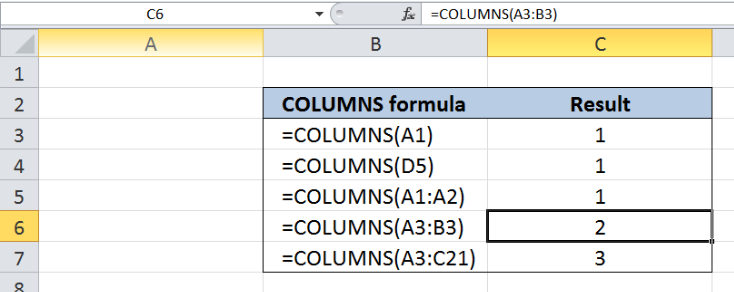Figure 6.  Output: COLUMNS function determines number of columns in a range

The COLUMNS function in Excel counts only the number of columns in any given array, regardless of the number of rows.

Most of the time, the problem you will need to solve will be more complex than a simple application of a formula or function. If you want to save hours of research and frustration, try our live Excelchat service! Our Excel Experts are available 24/7 to answer any Excel question you may have. We guarantee a connection within 30 seconds and a customized solution within 20 minutes.

### Did this post not answer your question? Get a solution from connecting with the expert.Another blog reader asked this question today on Excelchat:## Subscribe to Excelchat.coAnother blog reader asked this question today on Excelchat: